# Differential studies of inclusive J/$ψ$ and $ψ$(2S) production at forward rapidity in Pb-Pb collisions at $\mathbf{\sqrt{{\textit s}_{_{NN}}}}$ = 2.76 TeV

The production of J/$\psi$ and $\psi(2S)$ was measured with the ALICE detector in Pb-Pb collisions at the LHC. The measurement was performed at forward rapidity ($2.5 <~ y <~ 4$) down to zero transverse momentum ($p_{\rm T}$) in the dimuon decay channel. Inclusive J/$\psi$ yields were extracted in different centrality classes and the centrality dependence of the average $p_{\rm T}$ is presented. The J/$\psi$ suppression, quantified with the nuclear modification factor ($R_{\rm AA}$), was studied as a function of centrality, transverse momentum and rapidity. Comparisons with similar measurements at lower collision energy and theoretical models indicate that the J/$\psi$ production is the result of an interplay between color screening and recombination mechanisms in a deconfined partonic medium, or at its hadronization. Results on the $\psi(2S)$ suppression are provided via the ratio of $\psi(2S)$ over J/$\psi$ measured in pp and Pb-Pb collisions.

Figures

## Figure 1

 Fit to the opposite-sign dimuon invariant mass distribution in the 0-20$\%$ (upper row) and 40-90$\%$ (lower row) centrality classes, for $2.5 < y < 4$, in various $p_{\rm T}$ intervals.## Figure 2

 Fit to the opposite-sign dimuon invariant mass distribution after background subtraction in the 0-20$\%$ (upper row) and 40-90$\%$ (lower row) centrality classes, for $2.5 < y < 4$, in various $p_{\rm T}$ intervals.## Figure 3

 Opposite-sign dimuon invariant mass distribution for the 20-40$\%$, 40-60$\%$ and 60-90$\%$ centrality classes, for $2.5 < y < 4$ and $p_{\rm T} < 3$ Gev/$c$, before (top row) and after background subtraction (bottom row) via event mixing In these intervals the $\Psi$ signal is extracted whereas in all other centrality and $p_{\rm T}$ intervals, only the 95$\%$ confidence level upper limits are provided.## Figure 4

 The J/$\Psi$ acceptance times efficiency, shown as a function of centrality (left) and as a function of $p_{\rm T}$ and $y$, for the centrality class 0-90$\%$ (right). The vertical error bars in the left panel represent the statistical uncertainties.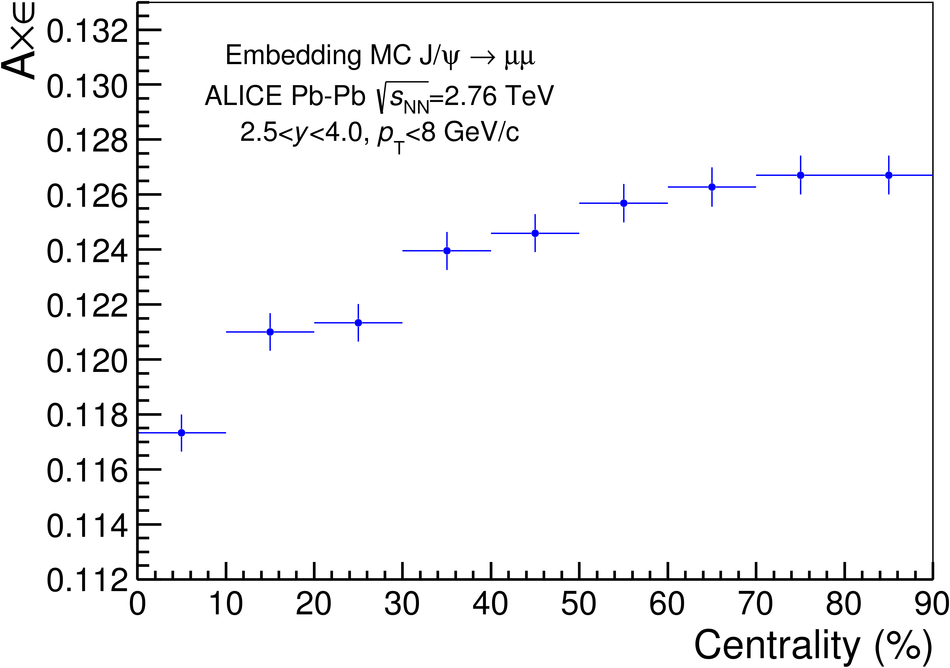## Figure 5

 Differential yields of inclusive J/$\Psi$ in Pb-Pb collisions at $\sqrt{S_{\rm NN}}=2.76$ TeV as a function of $p_{\rm T}$ for three centrality classes. Solid lines correspond to the results from the fit described in the text.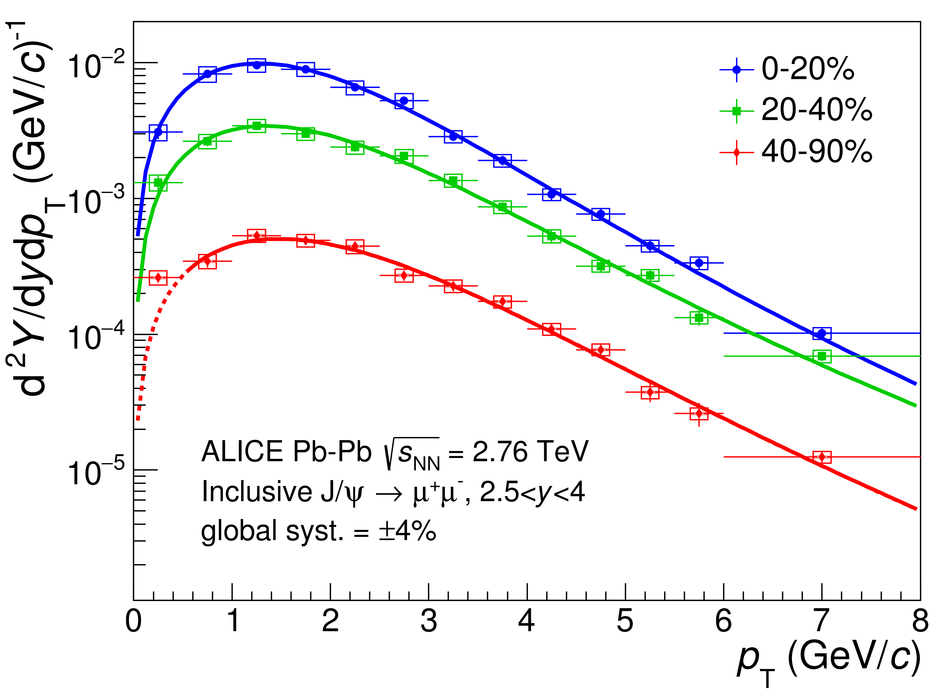## Figure 6

 Mean transverse momentum < $p_{\rm T}$> measured by ALICE and PHENIX as a function of the number of participant nucleons (left). $r_{\rm AA}$ measured by NA50, PHENIX and ALICE and compared to model calculations, as a function of the number of participant nucleons (right).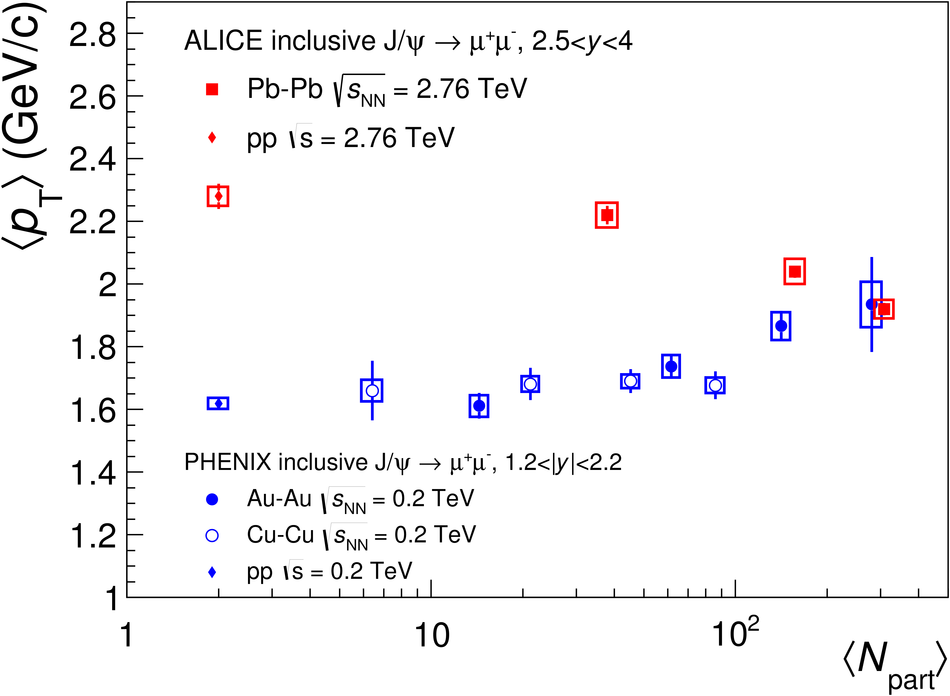## Figure 7

 Inclusive J/$\Psi$ $R_{\rm AA}$ as a function of the number of participant nucleons measured in Pb-Pb collisions at $\sqrt{s_{\rm NN}}=2.76$ TeV, compared to the PHENIX measurement in Au-Au collisions at $\sqrt{s_{\rm NN}}=0.2$ TeV (left) and to theoretical models, which all include a J/$\Psi$ regeneration component (right). The brackets shown in the three most peripheral centrality classes on the right figure quantify the possible range of variation of the hadronic J/$\Psi$ $R_{\rm AA}$ for two extreme hypotheses on the photo-production contamination in the inclusive measurement, see text for details.## Figure 8

 Inclusive J/$\Psi$ $R_{\rm AA}$ as a function of the number of participant nucleons measured in Pb-Pb collisions at $\sqrt{s_{\rm NN}}=2.76$ TeV for three $p_{\rm T}$ ranges (0-2, 2-5 and 5-8 Gev/$c$) and comparisons of the lowest and highest $p_{\rm T}$ range to the transport and to the comover interaction models. The brackets quantify the possible range of variation of the hadronic J/$\Psi$ $R_{\rm AA}$ for two extreme hypotheses on the photo-production contamination in the inclusive measurement.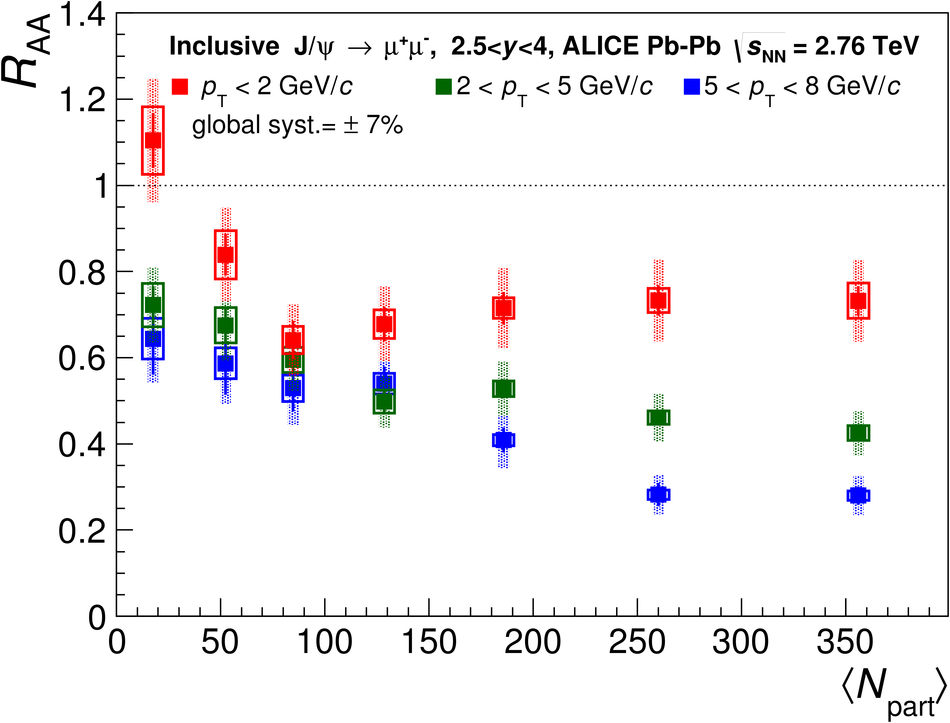## Figure 9

 Inclusive J/$\Psi$ $R_{\rm AA}$ as a function of the J/$\Psi$ $p_{\rm T}$ for $2.5 < y < 4$ in the centrality class 0-90$\%$ compared to transport models (top left). The comparison is done with PHENIX results and transport models in the 0-20$\%$ (top right), 20-40$\%$ (bottom left) and 40-90$\%$ (bottom right) centrality classes The brackets shown in the lowest $p_{\rm T}$ interval for the centrality class 40-90$\%$ quantify the possible range of variation of the hadronic J/$\Psi$ $R_{\rm AA}$ for two extreme hypotheses on the photo-production contamination in the inclusive measurement Upper limits from PHENIX at high $p_{\rm T}$ are not represented.## Figure 10

 Inclusive J/$\Psi$ $R_{\rm AA}$ as a function of the J/$\Psi$ rapidity measured in Pb-Pb collisions at $\sqrt{s_{\rm NN}}=2.76$ TeV, compared to theoretical calculations of CNM effects due to shadowing and/or coherent energy loss.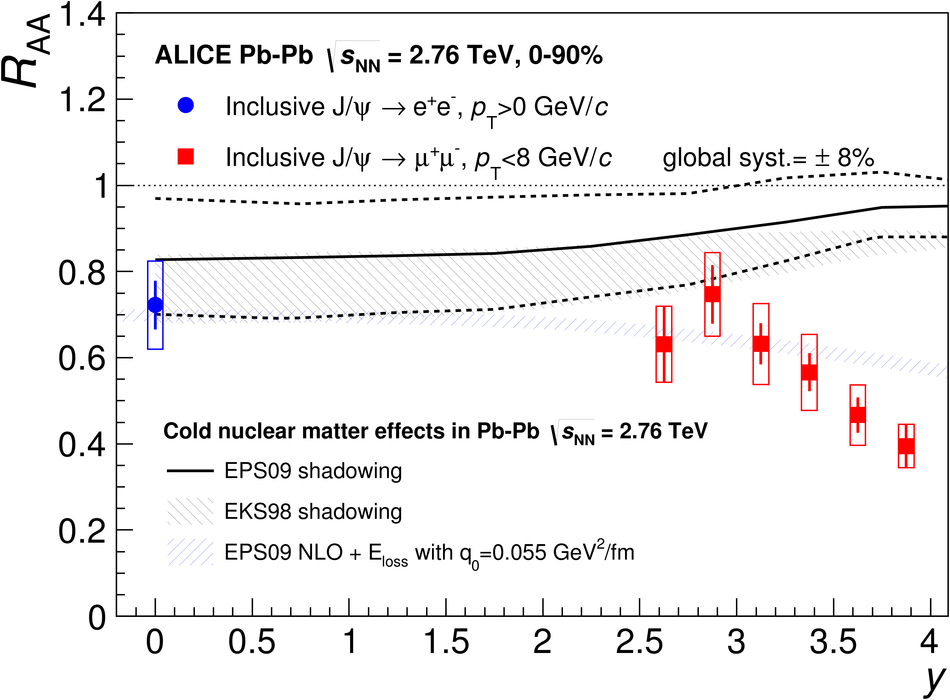## Figure 11

 Inclusive [$\Psi(\rm {2S})$/J/$\Psi$] ratio measured as a function of < $N_{\rm part}$> in Pb-Pb collisions at $\sqrt{s_{\rm NN}}=2.76$ TeV for two $p_{\rm T}$ intervals, compared to NA50 results and to a theoretical calculation (left) Double ratio, as a function of $N_{\rm part}$, between the $\Psi(\rm {2S})$ and J/$\Psi$ measured in Pb-Pb at $\sqrt{s_{\rm NN}}=2.76$ TeV and pp collisions at $\sqrt{s}=7$ TeV, compared to theoretical calculations (right).